# Independent Samples Z-Test - Comparing Two Proportions

Where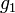$g_1$ and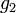$g_2$ are the two proportions and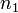$n_1$ and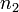$n_2$ are the sample sizes: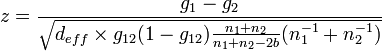$z=\frac{g_1-g_2}{\sqrt{d_{eff} \times g_{12}(1-g_{12})\frac{n_1+n_2}{n_1+n_2 - 2b}(n^{-1}_1+n^{-1}_2)}}$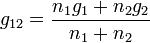$g_{12}=\frac{n_1 g_1 + n_2 g_2}{n_1+n_2}$,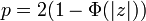$p = 2(1-\Phi(|z|))$,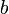$b$ is Bessel's correction, and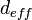$d_{eff}$ is Extra Deff.